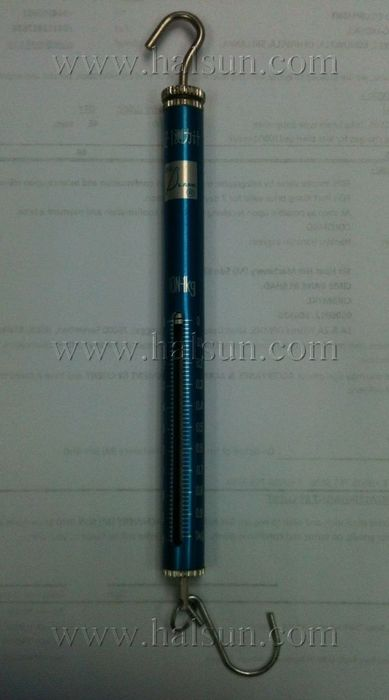# Newton Spring ScaleThe spring balance actually measures force, but for convenience is calibrated in kg on the basis that when it is used normally [weight force] = m * g where m is the mass in kg and g the acceleration due to gravity. (9.8 m/s^2)
So you just need to multiply the scale reading by 9.8 to get the force measurement in newtons.
1 kg reading => 9.8 N
2 kg reading => 19.6 N etc
Scales measure force…by definition
Balances measure mass.

Scales calibrated in kilograms are only done so for convenience, because we often use them to weigh in Earth standard gravity, and what we really want is the mass, but we cheat by using the scale instead of the balance.

Newtons aren’t a very popular unit in common use outside the study of physics…unfortunately, and so is the distinction of mass vs weight unpopular for one to understand.

For this reason, a 1 kg reading on a spring scale refers to 9.8 Newtons of tension, and it scales proportionally. This refers to the TENSION force with which the spring scale pulls on something.

If used to measure friction force, it is only done so indirectly. Because the target object is, in a good experiment, pulled at constant velocity, forces on it add up to zero, and as such, the tension need fully oppose the friction, should the scale be pulled horizontally and should the surface be flat.

#### You may have missed

```